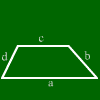# Trapezoid calculation

The following formulas are true for trapezoids:
Alpha + Delta = Beta + Gamma = 180░
Area = (a+c)/2*height

## What is a trapezoid?

A trapezoid is a quadrangle with two parallel sides. This is not saying much about the quadrangle, so it is quite difficult to do calculations. At least you know that angles lying at different of the parallel sides have the sum 180░.

## How can I imagine trapezoid formulas?

Most of them can be understood by cutting corners of the trapezoid and re-shaping it to a rectangle.

## What are the names of the sides and angles of a trapezoid?

Move the mouse over them and they are shown colored.Side a, Side b, Side c, Side d,
Angle alpha, Angle beta, Angle gamma, Angle delta,
Diagonal e, Diagonal f
Area
Height